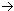Nuclear Binding Energy

The energy required to break down a nucleus into its component nucleons is called the nuclear binding energy.

63Cu + Energy29 p+ + 34 no

Nuclear binding energies are usually expressed in terms of kJ/mole of nuclei or MeV's/nucleon.  Calculation of the nuclear binding energy involves the following three steps:

Determining the Mass Defect

The difference between the mass of a nucleus and the sum of the masses of the nucleons of which it is composed is called the mass defect.  Three things need to be known in order to calculate the mass defect:

• the actual mass of the nucleus,
• the composition of the nucleus (number of protons and of neutrons),
• the masses of a proton and of a neutron.
To calculate the mass defect:
• add up the masses of each proton and of each neutron that make up the nucleus,
• subtract the actual mass of the nucleus from the combined mass of the components to obtain the mass defect.
Example:  Find the mass defect of a copper-63 nucleus if the actual mass of a copper-63 nucleus is 62.91367 amu.
• Find the composition of the copper-63 nucleus and determine the combined mass of its components.
• Copper has 29 protons and copper-63 also has (63 - 29) 34 neutrons.
The mass of a proton is 1.00728 amu and a neutron is 1.00867 amu.
The combined mass is calculated:

29 protons(1.00728 amu/proton) + 34 neutrons(1.00867 amu/neutron)
or
63.50590 amu

• Calculate the mass defect.
• Dm = 63.50590 amu - 62.91367 amu =  0.59223 amu

Conversion of Mass Defect into Energy

To convert the mass defect into energy:

• Convert the mass defect into kilograms (1 amu = 1.6606 x 10-27 kg)
• Convert the mass defect into its energy equivalent using Einstein's equation.
Example:  Determine the binding energy of the copper-63 atom.
• Convert the mass defect (calculated in the previous example) into kg.
(0.59223 amu/nucleus)(1.6606 x 10-27 kg/amu) = 9.8346 x 10-28 kg/nucleus
• Convert this mass into energy using DE = Dmc2, where c = 2.9979 x 108 m/s.
E = (9.8346 x 10-28 kg/nucleus)(2.9979 x 108 m/s)2 = 8.8387 x 10-11 J/nucleus

Top

Expressing Nuclear Binding Energy as Energy per Mole of Atoms, or as Energy per Nucleon

The energy calculated in the previous example is the nuclear binding energy.  However, nuclear binding energy is often expressed  as kJ/mol of nuclei or as MeV/nucleon.

• To convert the energy to kJ/mol of nuclei we will simply employ the conversion factors for converting joules into kilojoules (1 kJ = 1000 J) and for converting individual particles into moles of particles (Avogadro's Number).
(8.8387 x 10-11 J/nucleus)(1 kJ/1000 J)(6.022 x 1023 nuclei/mol) = 5.3227 x 1010 kJ/mol of nuclei
• To convert the binding energy to MeV (megaelectron volts) per nucleon we will employ the conversion factor for converting joules into MeV (1 MeV = 1.602 x 10-13 J) and the number of nucleons (protons and neutrons) which make up the nucleus.
(8.8387 x 10-11 J/nucleus)[1 MeV/(1.602 x 10-13 J)](1 nucleus/63 nucleons) = 8.758 MeV/nucleon